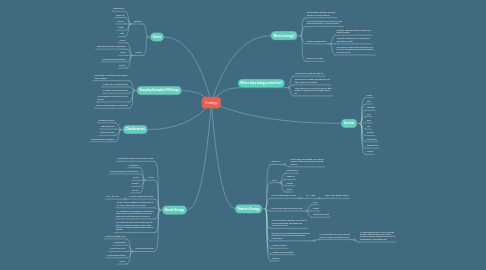# EnergyMohammed ALAUDDIN
Get Started. It's FreeEnergy## 1. What is energy?

### 1.3. Energy changes forms.

1.3.1. A toaster changes electrical energy into thermal energy.

1.3.2. A blender changes electric energy into mechanical energy.

1.3.3. Our bodies change chemical energy in our food into mechanical and electrical energy, so we can move.

## 3. Forms

### 3.1. Potential

3.1.1. Gravitational

3.1.2. Chemical

3.1.3. Nuclear

3.1.4. Elastic

3.1.5. Light

### 3.2. Kinetic

3.2.1. Motion

3.2.2. Thermal Energy and Temperature

3.2.3. Sound

3.2.5. Electric

## 7. Kinetic Energy

### 7.2. Types

7.2.1. Mechanical

7.2.2. Thermal Energy and Temperature

7.2.3. Sound

7.2.5. Electric

### 7.3. It can be measured in joules.

7.3.1. K.E. = 1/2 m v2

### 7.7. Real World Examples

7.7.1. A person pushing a box.

7.7.2. A dog barking

7.7.3. A hot cup of coco

7.7.4. A microwave working.

7.7.5. The TV.

## 8. Potential Energy

### 8.1. What is it?

8.1.1. Energy that is possessed by an object based on the position relative to other objects.

### 8.2. Types

8.2.1. Gravitational

8.2.2. Chemical

8.2.3. Nuclear

8.2.4. Elastic

### 8.3. It can be measured in joules.

8.3.1. P.E. = mgh

8.3.1.1. mgh= mass, gravity, height

### 8.4. Factors that affect potential energy.

8.4.1. Mass

8.4.2. Height

8.4.3. Gravitational force

### 8.6. Types of Forces that possibly exist between two objects which will be overcome by using energy.

8.6.1. All conservative forces, like gravity, elastic collisions and spring forces.

8.6.1.1. A conservative force is a force with the property that the total work done in moving a particle between two points is independent of the taken path.